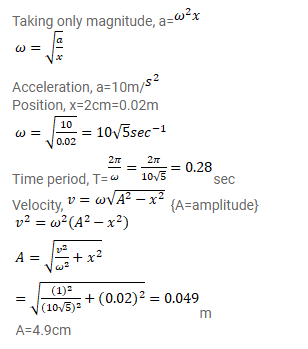# The position, velocity and acceleration of a particle executing simple

Question:

The position, velocity and acceleration of a particle executing simple harmonic motion are found to have magnitudes $2 \mathrm{~cm}$, $1 \mathrm{~m} / \mathrm{s}$ and $10 \mathrm{~m} / \mathrm{s}^{2}$ at a certain instant. Find the amplitude and the time period of the motion.

Solution: